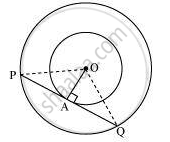# Two concentric circles of radii a and b (a > b) are given. Find the length of the chord of the larger circle which touches the smaller circle. - Mathematics

Sum

Two concentric circles of radii a and b (a > b) are given. Find the length of the chord of the larger circle which touches the smaller circle.

#### SolutionLet the two concentric circles be centered at point O.
Also, a > b where a is the radius of a larger circle and b is the radius of the smaller circle.
And let PQ be the chord of the larger circle which touches the smaller circle at point A. Therefore, PQ is tangent to the smaller circle.
OA ⊥ PQ (As OA is the radius of the circle)

Applying Pythagoras theorem in ΔOAP, we obtain

OA2+AP2=OP2

b2 + PA2 = a2

⇒  PA2 = a2 - b2

⇒ PA = sqrt("a"^2-"b"^2)

In ΔOPQ,

Since OA ⊥ PQ,

PA = AQ (Perpendicular from the center of the circle to the chord bisects the chord)

∴ PQ =2PA = 2sqrt("a"^2-"b"^2)

Therefore, the length of the chord of the larger circle is 2sqrt("a"^2-"b"^2) cm

Is there an error in this question or solution?
2018-2019 (March) All India (Set 2)

Share